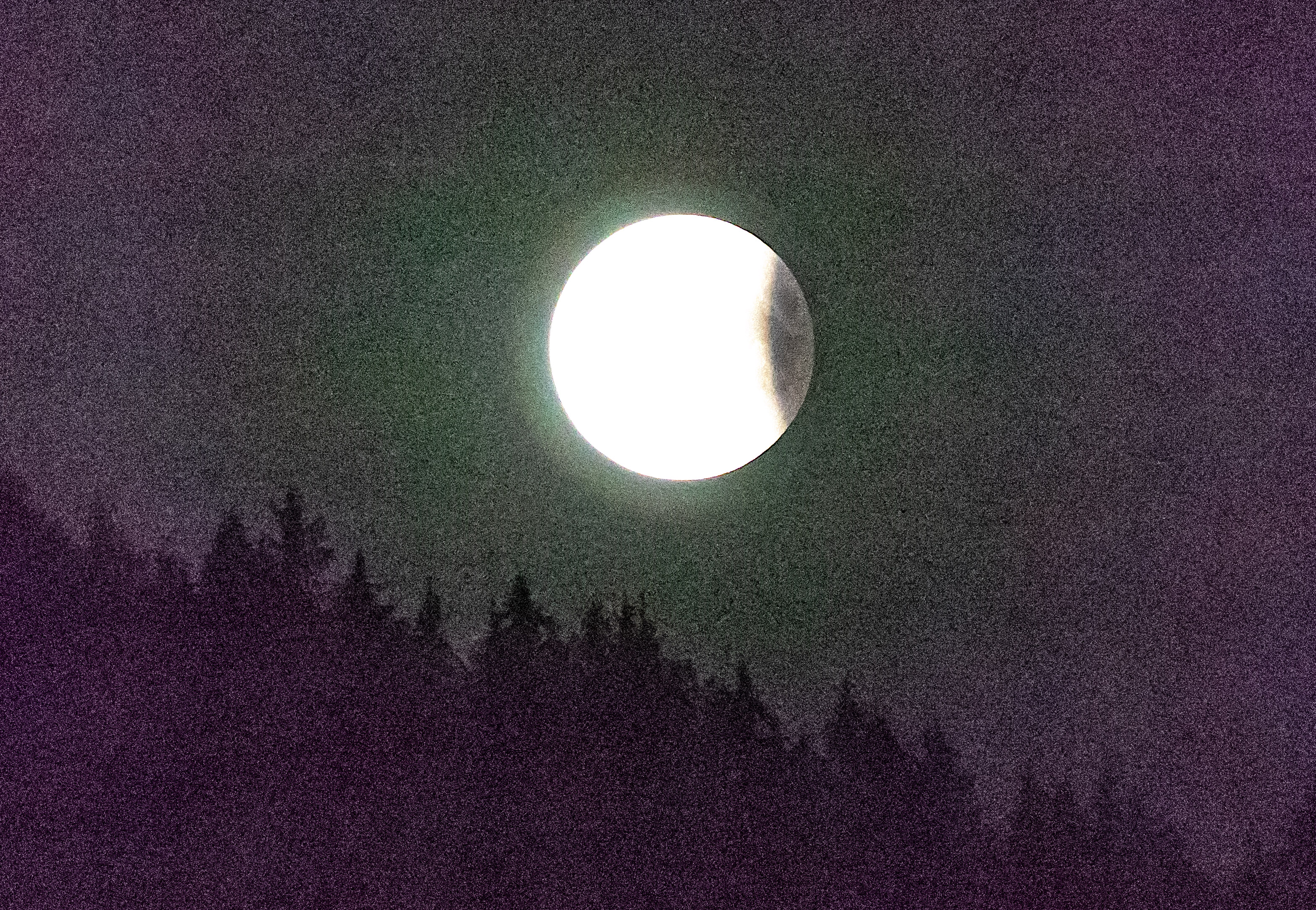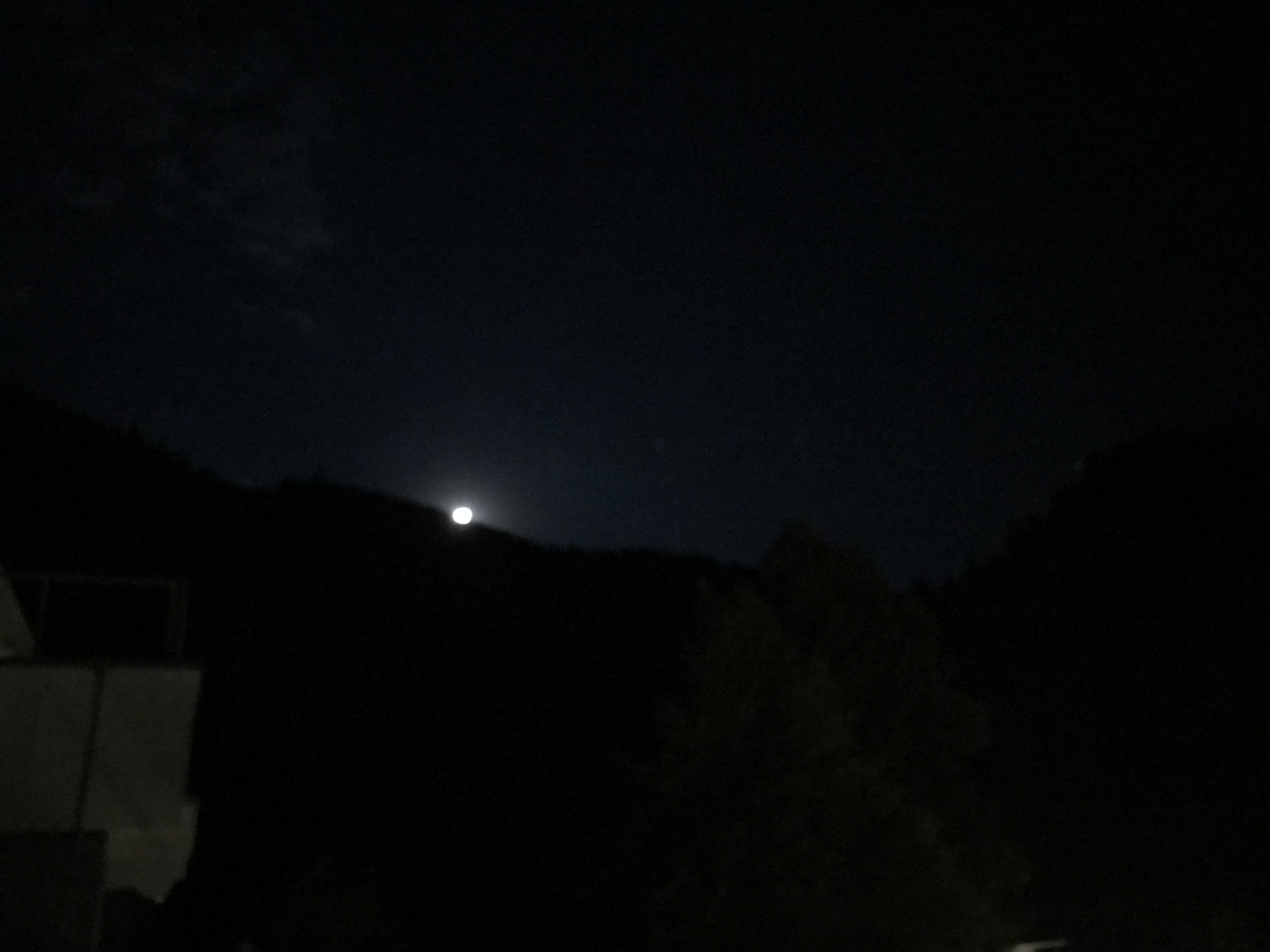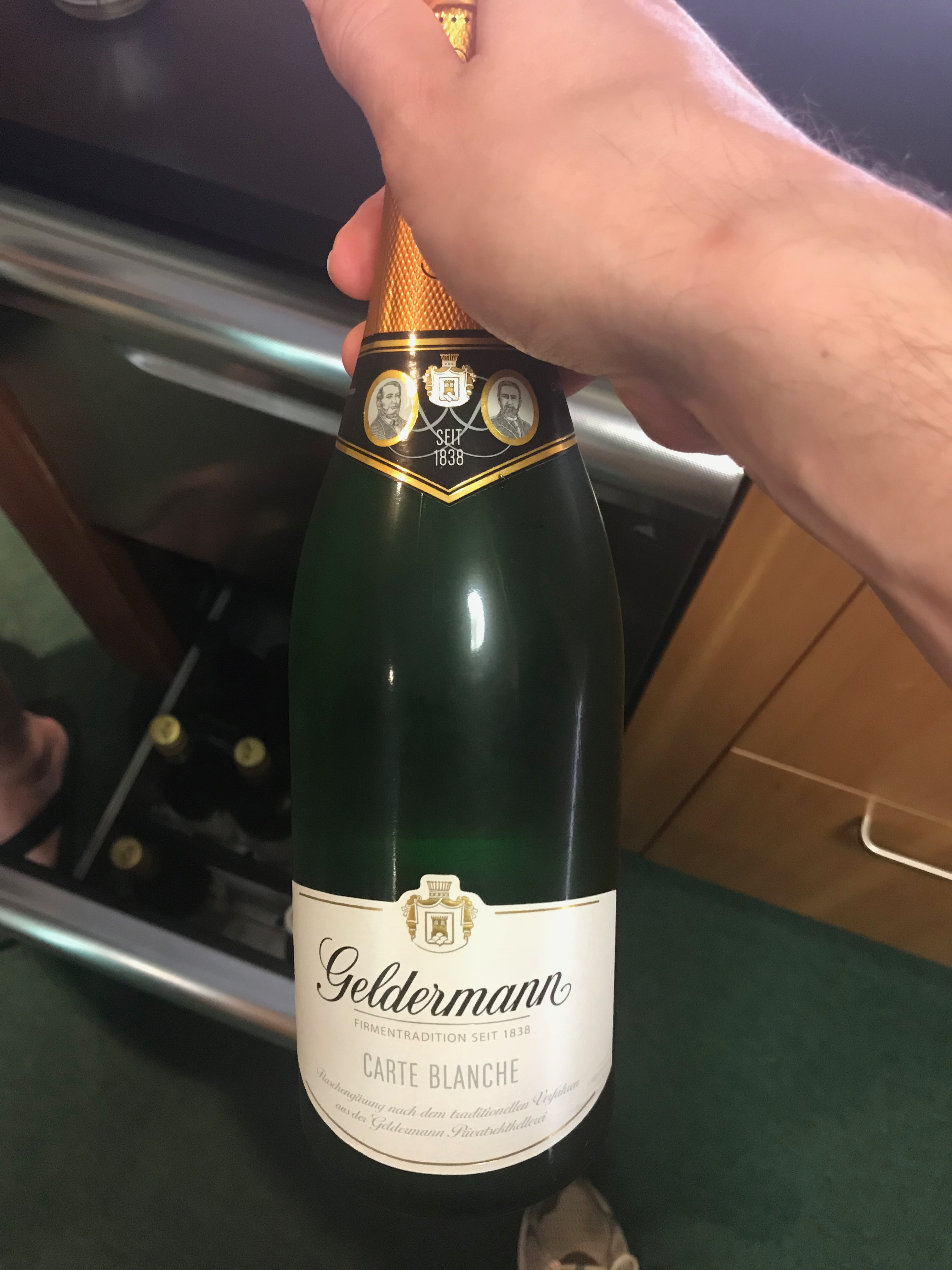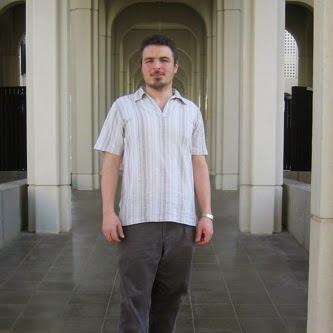## Mazur’s Program B on Abelian Surfaces

In the book “More mathematical people,” there is an interview with Robin Wilson with the following quote:

At the meal I found myself sitting next to Alistair Cooke who was very charming, and absolutely fascinating to listen to. The very next Sunday when I was back in England I turned on his “Letter from America” on the radio — he started off by saying, “I went to a very boring dinner at the White House. There was no one interesting to talk to.” That amused me a lot.

So let me start off by saying that even though this post is about one or two things I learnt at Oberwolfach, it is deliberately not about anything I learnt in the talks, lest my choosing some talks over others leading to false inferences on what I thought interesting. For example, the title of this post alludes to David Zureick-Brown’s talk, which I will not mention again.

Let g be a non-negative integer and p a prime. Suppose one starts with a representation$\displaystyle{\rho: G_{\mathbf{Q}} \rightarrow \mathrm{GSp}_{2g}(\mathbf{F}_p)}$

with (say) cyclotomic similitude character. To avoid later circumlocutions, let me (most of the time) assume it is absolutely irreducible. One can ask whether this representation arises infinitely often from the p-torsion on an abelian variety — perhaps additionally assuming that it does arise from at least one such variety, or perhaps not.

This problem is very well-studied in the case g = 1, where we know that the answer is positive exactly for the primes p = 2, 3, and 5, where the corresponding moduli space X(p) has genus zero, and the associated twists X(rho) are Brauer-Severi varieties that also turn out to be rational over Q under the given hypothesis on the similitude character. When p > 5, the curves X(p) have genus at least 3, and so their twists always have at most finitely many points over any field, by Faltings. So there is certainly a satisfactory answer in this case. (Of course, there are many more subtle versions of this question — for example, replacing “infinitely” by “at least twice” — and those variations are open in general.)

If we move to genus g = 2, then the case of p = 2 is also straightforward — the 2-torsion of the Jacobian of y^2 = f(x) for a degree 6 polynomial with Galois group G just comes from the isomorphism$G \hookrightarrow S_6 \simeq \mathrm{GSp}_4(\mathbf{F}_2).$ (One needs to be a little bit careful here because the outer automorphism of S_6 means there are two non-conjugate such maps and one has to choose the right one.) Given that one can write down families of sextics where the etale Q-algebra Q[x]/f(x) is constant, it’s easy to see that the answer is positive in this case without any restrictions. For example, given an$S_6$ extension, there’s a six dimensional family of polynomials one can write down whose splitting field generically gives this extension, and so after accounting for the action of$\mathrm{PGL}_2$ on the roots, this still gives a three-dimensional rational family of genus two curves whose two torsion comes from this extension.

In my paper with Boxer, Gee, and Pilloni (coming soon!), will also give a similarly conclusive answer for p = 3, although there are some unexpected surprises, as well as some complementary results recently proved by my student Shiva Chidambaram. But more on this in a post coming up soon!

When p > 3, then the corresponding 3-folds obtained by taking full level p-structure of the corresponding Siegel 3-fold$\mathcal{A}_2$ are of general type. (Note that it is essentially known when$\mathcal{A}_g(n)$ is either geometrically rational of general type, see for example Theorem II.2.1 and the surrounding comments in this paper.) Of course, unlike the case of curves, varieties of dimension greater than one of general type can have many rational points. For example, it’s obvious that there are many abelian surfaces over Q whose 5-torsion has the form$(\mathbf{Z}/5 \mathbf{Z} \oplus \mu_5)^2,$ because one can take A to be E + E where E is an elliptic curve whose 5-torsion has the form$(\mathbf{Z}/5 \mathbf{Z} \oplus \mu_5),$ and there are infinitely many such E because the classical modular curve of full level 5 is rational over Q. To put it a different way, the 3-fold A_2(5) corresponding to abelian surfaces with fixed 5-torsion will contain a number of rational Shimura subvarieties coming both from Hilbert modular surfaces and from modular curves, even though it itself is of general type. This can happen even if the mod-p representation rho is irreducible. For example, given an elliptic curve over a quadratic field K/Q, there will once more be a rational curve of elliptic curves with the same mod-5 representation, and so the restriction of scalars will give a rational curve on some twist A_2(rho) of A_2(5). On the other hand, one might at least start off by making the following naive minimal guess.

Question Suppose that$\rho$ is surjective for g = 2 and p >= 5. Then are there only finitely many points on$\mathcal{A}_2(\rho)?$

An even more extreme version of this question would be to ask if there is at most one such point. This seems a little unlikely even by comparison with the case of g=1. I learnt the following nice example talking to John Cremona during the hike through the Black Forest: for g=1 and p=7 and varying E, the twist X(E) has genus 3 (it is a twist of the Klein quartic). This twist is still geometrically a plane quartic. By considering the tangent to the point of X(E) corresponding to E, the line has two further intersections with the curve, and one obtains two further points over X(E) which now (in general) lie over a quadratic extension. But one can parametrize the E for which these points are actually *rational* and this turns out to be the rational cover of the$j$-line corresponding to asking that the invariant c_4 is a square. So there are infinitely many elliptic curves A (even with A surjective) for which there exist at least a pair of non-isogenous elliptic curves A,B with A=B as symplectic Galois representations. So a better question is the following:

Question Can one find examples of non-isogenous abelian surfaces A and B with A=B and such that the corresponding representation has a surjective Galois representation?

This is the type of question where it is useful to have Andrew Sutherland nearby with a laptop. Within an hour or two, he sent me the following examples:\displaystyle{\begin{aligned} C_1: y^2 = & \ -120x^6-264x^5+186x^4+276x^3-201x^2+24x \\ C_2: y^2 = & \ 16x^5 - 33x^4 + 60x^3 - 42x^2 + 36x - 9 \end{aligned}}

both of conductor$2^{10} \cdot 3^7$ with surjective and isomorphic mod-5 Galois representations which are not isogenous. Nice!

Naturally, the question turned to the existence of a pair with A = B. That proved a tougher challenge, but not an insurmountable one, and here is such a pair (again found by Andrew the same day):\displaystyle{\begin{aligned} C_1: y^2 + (x^3 + x) y = & \ -x^6+2x^4+2x^3+16x^2+4x+16 \\ C_2: y^2 + (x^2) y = & \ 14x^5-44x^4+46x^3-23x^2+12x-3 \end{aligned}}

this time of conductor$2^7 \cdot 3^2 \cdot 7^4.$

Any guesses as to whether there are any such pairs for$p = 11?$ I’m not sure I have any idea.

Other news from Oberwolfach:

I do appreciate being invited to the Oberwolfach conference on computational number theory — it pushes me outside my usual range of interests. It’s also the conference I have attended most often, now 8 times since 2003, although even that is far fewer than some of the regular participants. The conference is also chance to see a bunch of people I pretty much never get to see anywhere else. Even better, they are all nice enough to still invite me after this post. On the other hand, every time I give a talk I think that this is the time that I finally have something interesting to say to this audience, and it never quite seems to work out that way. I was certainly convinced that this was going to be the year, but then during my talk I managed to catch three people asleep in the front row. To be fair, it was the third last talk of the conference. On the other hand, Mike Bennett talked directly after me and completely failed to rise to my level of soporificness, despite his best efforts and his own predictions he would do otherwise.

There was a lunar eclipse on the final night of our stay. Most of us took to the roof to observe it, but the tall mountains of the Schwarzwald obscured our view until the final moment. Mike Bennett took the following photo, which he describes as the “best of a bad bunch”For comparison, here is my best photo of the same scene:Finally, if you are travelling to Oberwolfach during the summer, you mind will naturally turn to the question of whether you can enjoy the warm evenings by sipping on an Aperol spritz or two. Certainly they have lots of sparkling water, and so if Champagne (or equivalent) is available, you will easily be able to set yourself up simply by bringing along a bottle of Aperol. You might then consider trying to determine in advance whether they actually have any sparkling wine. Let me inform you, gentle reader, that the answer to that question is a definite yes, although you may have to look at the alcohol supply near the lecture theatre rather than near the dining room:Alas, my own intelligence was not up to snuff, as my informant on Facebook who was visiting the previous week was unable to locate this bottle.

There can be only one way to end this post (mostly only relevant to those brought up in Australia in the early 80s or perhaps in England a decade or so earlier:

Now for a walk in the Black Forest:

(10:40, 11:00, 18:52, 22:06 for relevant times if the timestamp link doesn’t work for you…)

### 2 Responses to Mazur’s Program B on Abelian Surfaces

1.Samir Siksek says:

Great post! Did you or Drew really verify the isomorphisms $A \cong B$, $A \cong B$ (e.g. using Faltings–Serre)? Or are they very plausible guesses based on matching thousands of characteristic polynomials of Frobenius modulo $5$, $7$?

2.galoisrepresentations says:

(very) Plausible guesses. My student Shiva Chidambaram has rigorously proved that $A \cong B$ for other pairs A and B by computing certain division polynomials on the Kummer surface quotient (so, for 5, you have to compare Galois groups of polynomials of degree (5^4-1)/2 = 312, something magma can handle). I’m not sure there’s any rigorous way to do it otherwise (the number of a_p one would have to check —- even using GRH forms of Cebotarev — would be impossibly large).